## MP Board Class 8th Maths Solutions Chapter 15 Introduction to Graphs Ex 15.2

Question 1.
Plot the following points on a graph sheet. Verify if they lie on a line
(a) A(4, 0), B(4, 2), C(4, 6), D(4, 2.5)
(b) P(1, 1), Q(2, 2), R(3, 3), S(4, 4)
(c) K(2, 3), L(5, 3), M(5, 5), N(2, 5).
Solution:
(a) A(4, 0), B(4, 2), C(4, 6), D(4, 2.5)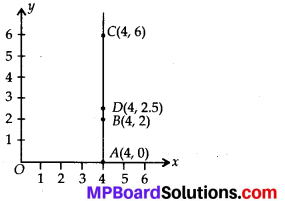Yes, above all points lie on the same line,

(b) P(1, 1), Q(2, 2), R(3, 3), S(4, 4).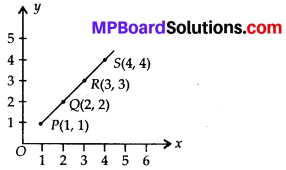Yes, above all points lie on the same line.

(c) K(2, 3), L(5, 3), M(5, 5), N(2, 5).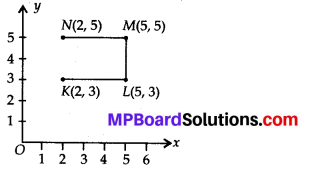No, above all points do not lie on the same line.

Question 2.
Draw the line passing through (2, 3) and (3, 2). Find the coordinates of the points at which this line meets the x-axis and y-axis.
Solution:
After drawing a graph the line passing through (2, 3) and (3, 2), then the co-ordinates of the points which meets the x-axis and y-axis are at (5, 0) and (0, 5) respectively.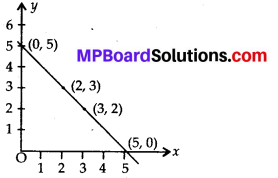Question 3.
Write the coordinates of the vertices of each of these adjoining figures.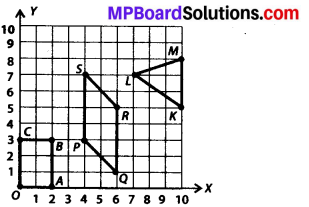Solution:
After observing a graph we find that O(0, 0), A(2, 0), B(2, 3), C(0, 3), P(4, 3), Q(6,1), R(6, 5), S(4, 7), L(7, 7), M(10, 8), K(10, 5).

Question 4.
State whether True or False. Correct that are false.
(i) A point whose x-coordinate is zero and y-coordinate is non-zero will lie on the y-axis.
(ii) A point whose y-coordinate is zero and x-coordinate is 5 will lie on y-axis.
(iii) The coordinates of the origin are (0, 0).
Solution:
(i) True
(ii) False, because it will lie on x-axis.
(iii) True.